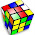## Thursday, February 22, 2007

### NPR Sunday Puzzle (Feb 24): As Easy as Pi...

NPR Sunday Puzzle (Feb 24): As Easy as Pi...:
Q: The object of this challenge is to develop nine different mathematical expressions that equal eight. You must use the digits 2, 7 and one other. And that other digit must be a one in the first expression, two in the next expression and so on, up to nine. You can use a digit once and only once in each expression.

You may use the four arithmetic symbols: plus, minus, times and divided by, as well as exponents and decimal points. You may use parenthesis as you need them.
Yippee! Another math puzzle! I like the word puzzles, but it's nice to have a math puzzle now and again. Unfortunately any clue I could provide would give away too much... just read the puzzle carefully and use all operators mentioned. As an extra challenge, see if you can make eight from 2, 7 and 0 also.

A: Here are my answers. As mentioned, I found I had to use the four basic operators and exponent and decimal too.
ONE: 7 + 2 - 1 = 8
TWO: 7 + ( 2 / 2 ) = 8
THREE: 7 + 3 - 2 = 8
FOUR: 2^( 7 - 4 ) = 8
FIVE: 7 + ( 2 * .5) = 8
SIX: (2 * 7) - 6 = 8
SEVEN: ( 7 / .7 ) - 2 = 8
EIGHT: 7 + .2 + .8 = 8
NINE: (7 + 9) / 2 = 8
ZERO: 7 + 2^0 = 8

1.2.